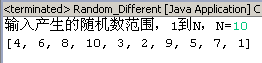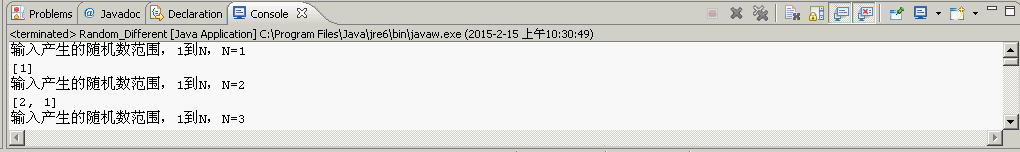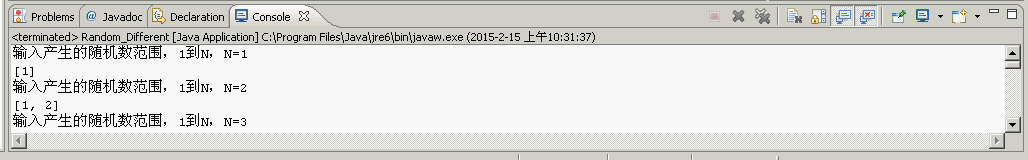【Java】在一定的范围内产生不同的随机数

import java.util.*;

public class Random_Test {
public static void main(String[] args) {
System.out.println(new Random().nextDouble());//能产生一个0-1的随机小数
System.out.println(new Random().nextInt(10));//能产生一个大于或等于0，小于但不等于10的随机整数，也就是个位数
}
}

import java.util.*;
import java.io.*;

public class Random_Different {
public static void main(String[] args) throws IOException{
System.out.print("输入产生的随机数范围，1到N，N=");
int n=0;
try{
}catch(Exception e){
System.out.println("N必须为正整数");
return;
}
if(n<1){
System.out.println("N必须为正数");
return;
}
int randArr[]=new int[n];
int i=0;
while(i<n){
int rand=(new Random().nextInt(n)+1);
boolean isRandExist=false;
for(int j=0;j<randArr.length;j++){
if(randArr[j]==rand){
isRandExist=true;
break;
}
}
if(isRandExist==false){
randArr[i]=rand;
i++;
}
}
System.out.println(Arrays.toString(randArr));
}
}